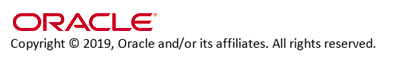Calculate Freight by Order Weight for N/C Orders (G68)

Purpose: Use this screen to define whether the system charges freight for orders whose merchandise total is zero, when you calculate freight by order weight.

Yes/No field: Select this field if you wish the system to charge freight for orders whose merchandise total is zero, when you calculate freight by order weight.

You may want to charge freight on an order whose merchandise total is zero, if the order includes a warranty item.

The system calculates freight on the order by order weight if the source code defined on the order header is set up to calculate freight using the order weight freight method. See Working with Weight Tables for Order Weight (WFTB), for more information on how the system calculates freight by order weight.

Leave this field unselected if you do not want the system to charge freight for orders whose merchandise total is zero, when you calculate freight by order weight.

The system only charges freight for orders whose merchandise total is greater than zero.IN03_02 OMSCS 19.0 December 2019 OHC